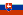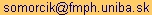# Ján Somorčík

University
Faculty
Department
Division## Teaching

• Statistical Methods
• Nonparametric Statistics
• Computer Statistics
• Econometrics
• Probability Theory (exercises)

## Research

• Robust location and scatter matrix estimators:
• Somorčík J. (2006): Tests Using Spatial Median. Austrian Journal of Statistics, Vol. 35, No. 2&3, 331-338. (pdf)
• Somorčík J. (2007): Performance of Some Spatial Median Tests under Elliptical Symmetry. Measurement Science Review, Vol. 7, Section 1, No. 4, 43-50. (pdf)
• Somorčík J., Rublík F. (2009): Multi-Sample Spatial Median Test Not Requiring Distributions of the Same Type. In: Sborník prací 15. letní školy JČMF ROBUST 2008 (eds. Antoch, J., Dohnal, G.), JČMF, Praha, 385-392. (pdf)
• Rublík F., Somorčík J. (2011): Affine-Equivariant Spatial Median and Its Use in the Multivariate Multi-Sample Location Problem. Australian & New Zealand Journal of Statistics, Vol. 53, No. 2, 197–215. (pdf)
• Rublík F., Somorčík J. (2015): On the Behrens–Fisher problem from the spatial median point of view. Journal of Statistical Computation and Simulation, Vol. 85, No. 15, 3080-3091. (pdf)
• Tóth R., Somorčík J. (2017): On a non-parametric confidence interval for the regression slope. METRON, Vol. 75, No. 3, 359–369. (pdf)

## Contact

•• Department of Applied Mathematics and Statistics
Faculty of Mathematics, Physics and Informatics
Comenius University
Mlynská dolina
842 48 Bratislava 4
Slovak Republic
• Office: M 245